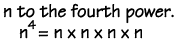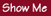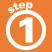Home    |    Teacher    |    Parents    |    Glossary    |    About UsIn algebra, you'll often be working with exponents. Here are some rules:Any number raised to the zero power (except 0) equals 1.Any number raised to the power of one equals itself.To multiply terms with the same base, add the exponents.To divide terms with the same base, subtract the exponents.When a product has an exponent, each factor is raised to that power.A number with a negative exponent equals its reciprocal with a positive exponent.Homework Help | Algebra | The Basics of AlgebraEmail this page to a friendSearch·  Properties of     Real Numbers·  Exponents·  Evaluating expressions·  Like terms·  SimplifyingFirst Glance In Depth Examples WorkoutExponents